A transformer is an electrical device used for converting low alternating voltage into a high alternating voltage and vice versa. It transfers electric power from one circuit to another. The transformer is based on the principle of electromagnetic induction.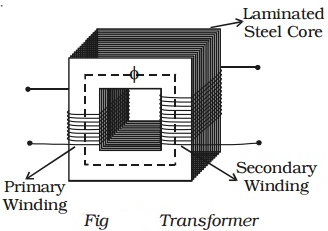A transformer consists of primary and secondary coils insulated from each other, wound on a soft iron core (Fig 4.15). To minimize eddy currents a laminated iron core is used. The a.c. input is applied to the primary coil. The continuously varying current in the primary coil produces a varying magnetic flux in the primary coil, which in turn produces a varying magnetic flux in the secondary. Hence, an induced emf is produced across the secondary.

One of the coils called the primary coil has Np turns. The other coil s called the secondary coil; it has Ns turns. Often the primary coil is the input coil and the secondary coil is the output coil of the transformer. Since same flux links with the primary and secondary, the emf induced per turn of the two coils must be the same.

Let ϕ be the flux in each turn in the core at time t due to the current in the primary when a voltage vp is applied to it.

Then the induced emf or voltage , in the secondary with Ns turns is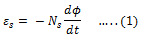The alternating flux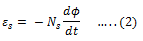But εp = vp . If this were not so, the primary current would be infinite since the primary has zero resistance (as assumed). If the secondary is an open circuit or the current taken from it is small, then to a good approximation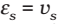where vs is the voltage across the secondary. Therefore, Eqs. (1) and (2) can be written as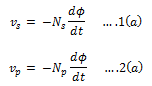From eqs. 1(a) and 2(a), we haveNote that the above relation has been obtained using three assumptions: (i) the primary resistance and current are small; (ii) the same flux links both the primary and the secondary as very little flux escapes from the core, and (iii) the secondary current is small.

If the transformer is assumed to be 100% efficient (no energy losses), the power input is equal to the power output, and since p = iv,Combining eqs. (3) and (4), we have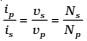Since i and v both oscillate with the same frequency as the ac source.

Now, we can see how a transformer affects the voltage and current. We have: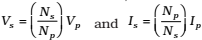### Types of Transformer

There are two types of the transformer and they are:

1. Step-Up Transformer
2. Step-Down Transformer

### Step-Up transformer:

if the secondary coil has a greater number of turns than the primary (Ns > Np), the voltage is stepped up (Vs > Vp). This type of arrangement is called a step-up transformer. However, in this arrangement, there is less current in the secondary than in the primary (Np/Ns < 1 and Is < Ip).

### Step-Down transformer:

If the secondary coil has less turns than the primary (Ns < Np), we have
a step-down transformer. In this case, Vs < Vp and Is > Ip. That is, the voltage is stepped down, or reduced, and the current is increased.

### Efficiency of a transformer

The efficiency of a transformer is defined as the ratio of output power to the input power.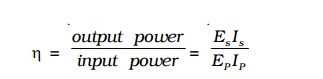The efficiency η = 1 (ie. 100%), only for an ideal transformer where there is no power loss. But practically there are numerous factors leading to energy loss in a transformer and hence the efficiency is always less than one.

### Energy losses in a transformer

#### Hysteresis loss:

The magnetization of the core is repeatedly reversed by the alternating magnetic field. The resulting expenditure of energy in the core appears as heat and is kept to a minimum by using a magnetic material which has a low hysteresis loss.

#### Flux Leakage:

There is always some flux leakage; that is, not all of the flux due to primary passes through the secondary due to poor designs of the core or the air gaps in the core. It can be reduced by winding the primary and secondary coils one over the other.

#### The resistance of the windings:

The wire used for the windings has some resistance and so, energy is lost due to heat produced in the wire (I2R). In the high current, low voltage windings, these are minimized by using thick wire.

#### Eddy currents:

The alternating magnetic flux induces eddy currents in the iron core and causes heating. The effect is reduced by having a laminated core.